# NCERT Solutions - Chapter 4 - Theory of Firm Under Perfect Competition, Class 12, Economics Humanities/Arts Notes | EduRev

## Humanities/Arts : NCERT Solutions - Chapter 4 - Theory of Firm Under Perfect Competition, Class 12, Economics Humanities/Arts Notes | EduRev

The document NCERT Solutions - Chapter 4 - Theory of Firm Under Perfect Competition, Class 12, Economics Humanities/Arts Notes | EduRev is a part of the Humanities/Arts Course NCERT Textbooks (Class 6 to Class 12).
All you need of Humanities/Arts at this link: Humanities/Arts

NCERT Solutions for Class 12th Microeconomics

Chapter 4 – Theory of Firm

Question 1. What are the characteristics of a perfectly competitive market?

Answer The main characteristics of a perfectly competitive market are as follows
(i) Large number of buyers and sellers.
(ii) Homogeneous goods
(iii) Free entry and exit of firms.
(iv) Buyers and sellers have perfect knowledge of market.
(v) Perfect mobility of factors of production.
(vi) The transportation costs assumed zero.
(vii) There is no selling costs.

Question 2. How are the total revenue of a firm, market price and the quantity sold by
the firm related to each other?

Answer Total Revenue is the sum total of revenue receipts from the sale of a given
quantity of a commodity. It means total revenue is obtained by multiplying the market
price (sale price) of commodity and quantity of the commodity sold.
Total Revenue = Market price x Quantity sold

Question 3. What is the ‘price line’?

Answer It is a horizontal line that represents the market price for a perfectly competitive
firm and output Under monopoly of monopolistic &. competition, firm price line slope
downward For a perfectly competitive firm, price line and demand curve are same

Question 4. Why is the total revenue curve of a price-taking firm an upward sloping
straight line? Why does the curve pass through the origin?

Answer For a price taking firm, AR is constant. In case AR is constant, MR is also
constant. As a result TR increases in the same proportion as price is constant. So, TR
curve is upward sloping straight line. It passes from the origin because TR is zero
at zero level of output.

Question 5. What is the relation between market price and average revenue of a pricetaking firm?

Answer For a price taking firm, market price is equal to average revenue.
We know, AR=TR/Q
TR= P x Q, AR =P x Q/Q
AR= P
Here AR=Average Revenue
TR = Total Revenue

Question 6. What is relation between market price and marginal revenue of a pricetaking
firm?

Answer For a price taking firm, market price is equal to marginal revenue because firm
can sell more quantity of commodity at the same price. As a
result that revenue from every additional unit (MR) is equal to price or average revenue
AR.

Question 7. What conditions must hold if a profit maximising firm produces positive
output in a competitive market?

Answer When price remains constant firms can sell any quantity of output at the price
fixed by the market. AR remains same at all levels of output and also revenue from
every additional unit (MR) is equal to AR It means, AR curve is same as MR
curve. Producer aims to produce that level of output at which Me is equal to MR and Me
is greater than MR after MC = MR output level.

Question 8. Can there be a positive level of output that a profit maximising firm
produces in a competitive market at which market price is not equal to marginal cost?
Give an explanation.

Answer No, because it is not possible as equality between market price and marginal
cost is a necessary condition for perfectly competitive firm to be in equilibrium. Only
when price remain constant at all output levels, the market price is equal to marginal
revenue in case of perfect competition.

Question 9. Will a profit maximising firm in a competitive market ever produce a
positive level of output in the range where the marginal cost is falling? Give an
explanation.

Answer No, because the essential condition of producer’S equilibrium is that marginal
cost curve should be rising So. a profit maximising firm will
produce that quantity of output at which its MC is rising and not falling.

Question 10. Will a profit maximising firm in a competitive market produce a positive
level of output -in the short run if the market price is less than the minimum of AVC ?
Give an explanation.

Answer No, a profit maximising firm will not produce a level of output in the short run
when market price is less than the minimum of AVC. It
happens because equality between market price and minimum AVC indicates shut
down point and a firm will never operate at a price less than the minimum AVC.

Question 11. Willa profit maxximising firm in a competitive market produce a positive
level of output in the short run if the market price
is less than the minimum of AC?Give an explanation.

Answer No, it is not possible for a firm to produce positive level of output in the long
run, if the market price tails short of the minimum of AC. It is
because in long run there is free entry and exit of firms which leads to generate normal
profit as their earning. Thus. any firm making loss in long
fun slop the production

Question 12. What is the supply curve of a firm in the short run?

Answer The supply curve of a firm in the short run is less elastic and it is responsive to
changes in price.

Question 13. What is the supply curve of a firm in the long run?

Answer The supply curve of a firm in the long run Is highly elastic and it is more
responsive to change in price.

Question 14. How does technological progress affect the supply curve of a firm?

Answer The technological progress affect the supply curve of a firm will shuts to
downward (to the right) Because a firm can produce same level of
output using less of inputs with improved technology. It causes fall to the marginal cost.

Question 15. How does the imposition of a unit tax affect the supply curve of a firm?

Answer A unit tax may be defined as the tax imposed by the government on per unit
sale of output. The imposition of a unit tax shifts the marginal
cost curve of the firm upward Affect in supply curve will shift to the left.

Question 16. How does an increase in the price of an input affect the supply curve of a
firm?

Answer An increase in the price of an input will affect marginal cost curve upward. So,
supply curve shifts to the left. Therefore, an Increase in the
input price negatively affects the supply of the firm.

Question 17. How does an increase in the number of firms in a market affect the
market supply curve?

Answer If number of firms increase in a market, the market supply curve will shift to the
right as there will be more number of firms supplying more
amount of output.

we measure it?
Answer The price elasticity of supply means the percentage change in
quantity supplied caused by a given percentage change in price ot commodity,
It is measured as
Price elasticity of supply (Es) = Percentage of quantity supplied/ percentage change in
price
Es= ΔQ/ΔP x P /Q
ΔQ= change in supply
Δp= change in price
p= initial price
Q= initial supply

Question 19. Compute the total revenue, marginal revenue and average revenue
schedules for the following Market price of each unit of the good is Rs 10.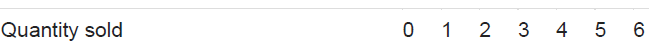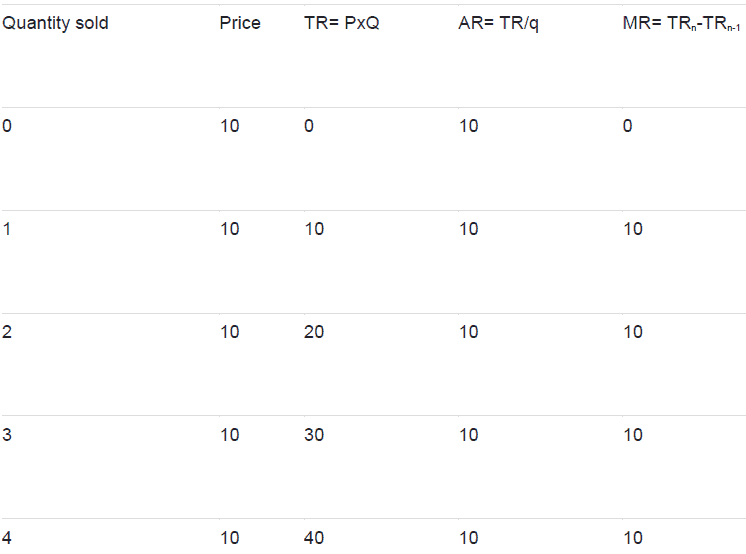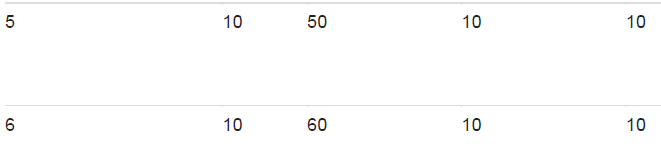Question 20. The following table shows the total revenue and total cost schedules of a
competitive firm. Calculate the profit at each output level. Determine also the market
price of the good.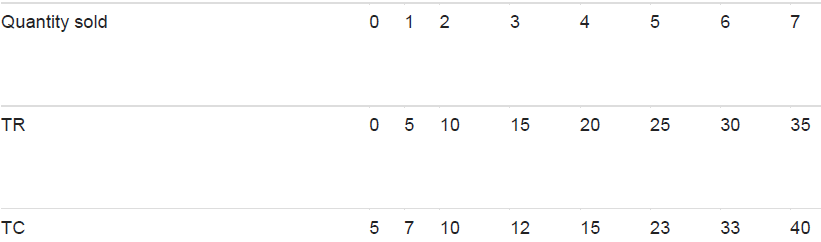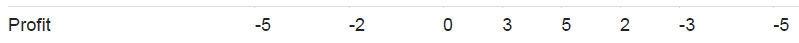Market price=TR/Q
i.e., 5/1 =5 at 2nd stage
10/2= 5 in 3rd stage
and so on

Question 21. The followinq table shows the total cost schedule of a competitive firm. It
is given that the price of the good is Rs 10. Calculate the profit at each output level. Find
the profit maximising level of output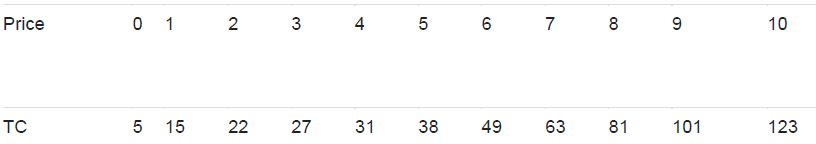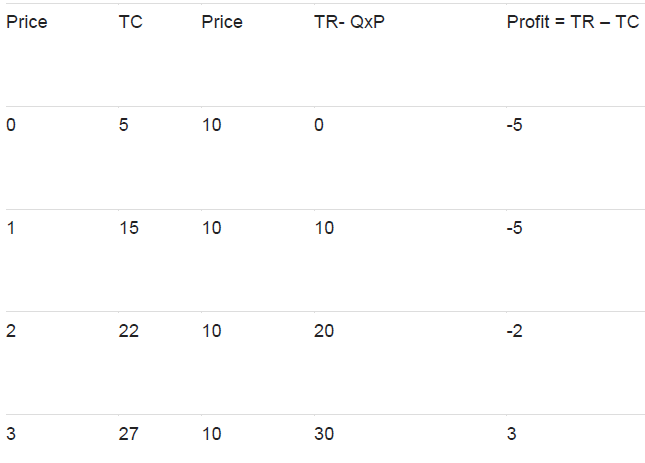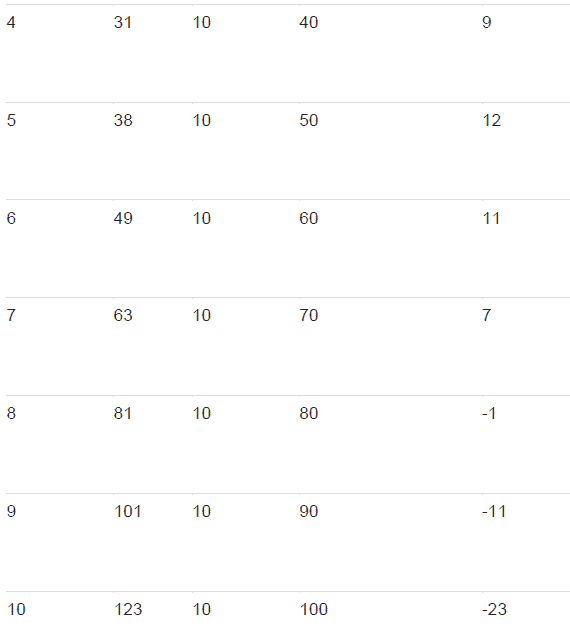The profit maximising level at 5 units sold where firm is earning profit of Rs 12.

Question 22. Consider a market with two firms. The following table shows the supply
schedules of the two firms; SS1 column gives the supply of firm schedule 1 and the
SS2 column gives the supply schedule of firm 2. Compute the market supply schedule .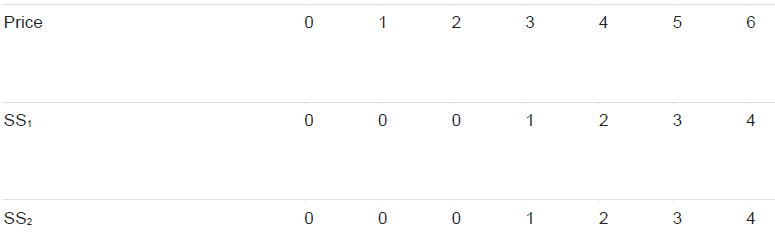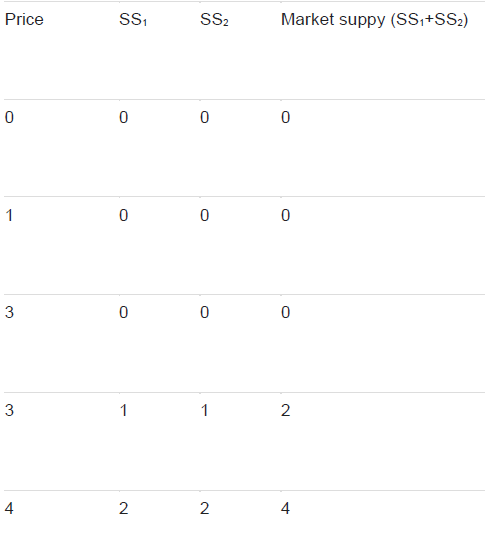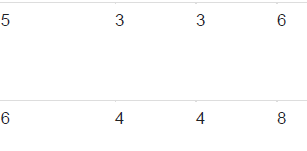Question 23. Consider a market with two firms. In the following table, columns labelled
as SS1 and SS2 give the supply schedules of firm 1 and firm 2 respectively. Compute the
market supply schedule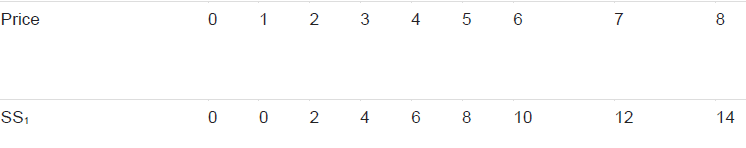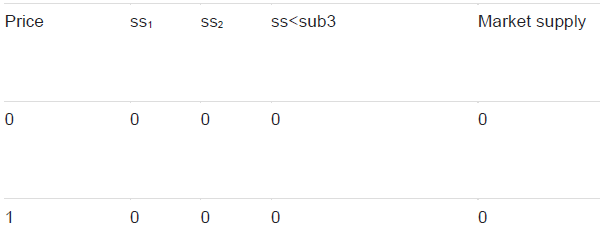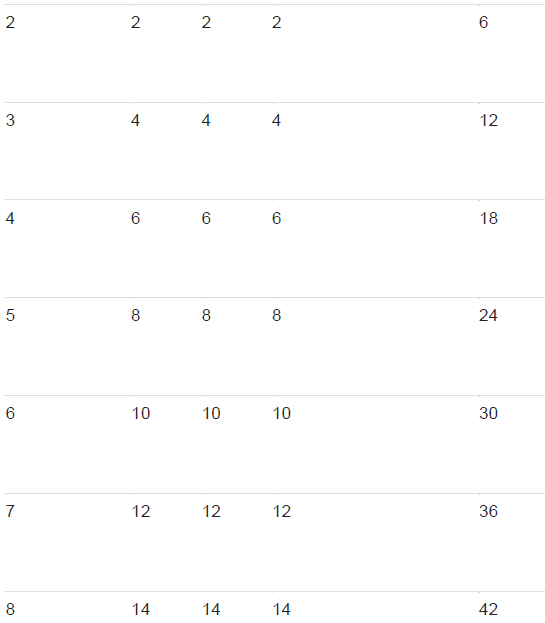Explanation If the three firms are identical, supply of the each firm will be equal.

Question 25. A firm earns a revenue of Rs 50 when the market price of a good is ~ 10.
The market price increases to Rs 15 and the firm now earns a revenue of Rs 150. What
is the price elasticity of the firm’s supply curve?

Answer (i) When market price (p) =10
Revenue = Rs 50
Quantity supplied (Q) = 50/10 = 5 units

(ii) When market price (P1) =15
Revenue = Rs150
Quantity supplied (Q1) 150/15 =10units
Now given P= 10 , P1 = 15
Q= 5 units
Q1= 10units
Change in Price (ΔP):: 15-10 =Rs5
Change in Quantity (ΔQ) = 10- 5 = 5 units
Price elasticity of supply EsΔP/ΔQ x P/Q
5/5 x 10/5
Es = 2 (elastic supply)

Question 26. The market price of a good changes from Rs 5 to Rs 20. As a result, the
quantity supplied by a firm increases by 15 units. The price elasticity of the firm’s supply
curve is 0.5. Find the initial and final output levels of the firm.

New Price (P1) C = Rs 15, ΔQ= 15 units
Elasticity of supply (Es) = 0.5
Price elasticity of supply (Es) = ΔQ/ΔP x P/Q
0.5 = 15/15 x 5/Q
Initial Output (0) =10 units
Final Output = 10 units + 15 units= 25 units

Question 27. At the market price of ~ 10, a firm supplies 4 units of output. The market
price increases to ~ 30. The price elasticity of the firm’s supply is 1.25. What quantity
will the firm supply at the new price?

Q= 4 units
P=Rs 10, P1 = Rs30
Change in price (ΔP) = Rs20 (P-P1)
Es=1.25
Es= ΔQ/ΔP x P/Q= 1.25=ΔQ/20 x 10/4
ΔQ= 10 units
Quantity at new price = 10+ 4 = 14 units

Offer running on EduRev: Apply code STAYHOME200 to get INR 200 off on our premium plan EduRev Infinity!

## NCERT Textbooks (Class 6 to Class 12)

954 docs|585 tests

,

,

,

,

,

,

,

,

,

,

,

,

,

,

,

,

,

,

,

,

,

,

,

,

,

,

,

;